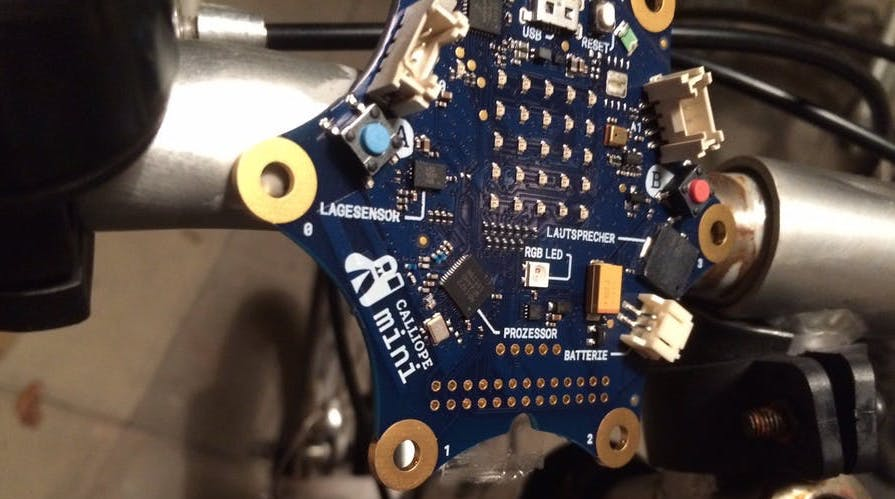# Speedometer

## Story

Measuring speed with the Calliope mini
With classic speedometers, speed is measured using a sensor on the frame and a magnet on a spoke. Each time the magnet passes the sensor, the bike computer measures the time and calculates the speed using the circumference of the wheel (which you have to set in the bike computer).

## INSTRUCTIONS

Step 1
In this Calliope project we will simulate the sensor with the button A.
First, we need to set the wheel circumference to 2200mm at the start of the program, which is about the circumference of a typical bikewheel:

Step 2
We want the speed to be calculated when pressing button A. For this, we will memorize the current running time in the placeholder "new_time". The running time is the number of milliseconds that have passed since the program started.

Step 3
When button A is pressed for the first time, "last_time" is still 0, because we set this placeholder according to the calculation to the currently measured time. So we can only perform the calculation if "last_time" is greater than 0:

Step 4
In order to be able to calculate the speed, we now still need the passed time. For that we simply need to subtract the time measured with the last press of A from the just measured time:

Step 5
Now we are left with the time interval in milliseconds between the current and the last measurement in the placeholder "time_diff". We can now calculate the speed if we divide the distance covered by the passed time (volume/time_diff). Since the unit mm/ms is rarely ever used, we convert it to km/h by multiplying it by 3.6. Since decimal numbers are not supported, and to get the best possible accuracy, we multiply it by 36 and divide it by 10 at the end.

Step 6
Now we have stored the speed in km/h in the placeholder "speed". We want it as output on the LED matrix. Since the direct output of numbers does not work, we have to use an old JavaScript trick and convert the number into a string by "adding" it with an empty string.

Step 7
Since we now notice the last measured speed is displayed forever, although we are not keeping the button pressed anymore, we add a recognition for the speed "0". We have to check if more than 2 seconds have passed since the last measurement. If so, we set speed to 0.

## Schematics

This text as well as the image is published under a CC BY-SA 3.0 DE license. It was originally published in German popup: yes by Daniel Havlik popup: yes and translated into English by the Calliope team.

hex